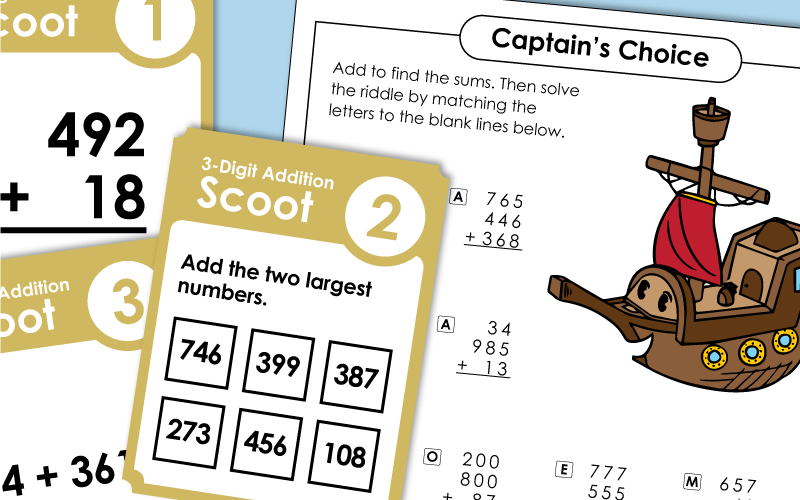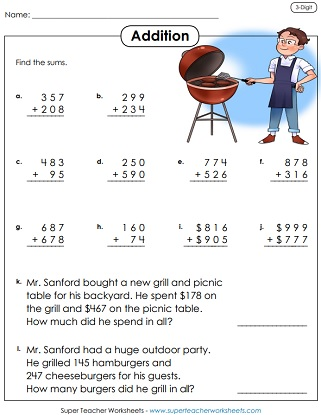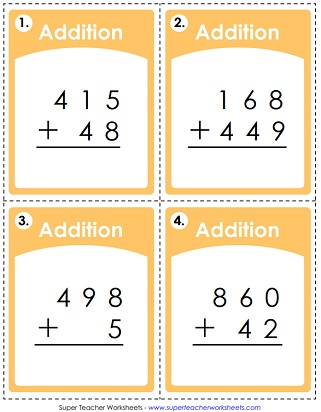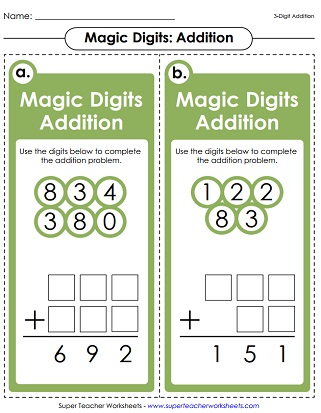Add the 3-digit numbers starting with the ones, then tens, then hundreds. Boxes are available to show regrouping/carrying.
Solve this set of addition problems with 3-digit addends. Includes two word problems.
Use this worksheet to help students practice adding with three digits.
Find the sum for each problem. All addition requires carrying or regrouping. Use the answers to solve the riddle: What's more amazing than a talking dog?
In this game, students in your class will travel to each desk and answer addition problems. All problems have at least one 3-digit addend.
This file has 30 addition task cards. Each one has a problem with 3-digit addends. There's also a student answer worksheet. Makes a great learning center.
Add numbers inside similar shapes. For example: Add the numbers inside the hexagons.
These math problems have mistakes in the solutions. Write the correct solution, and explain why the problems shown are not correct.
First, solve the 3-digit addition problems. Then cut out the cards. Sort the sums into two groups: even and odd. Glue the cards on a t-chart.
Kids practice adding three-digit numbers; Requires Carrying / Regrouping.2,
Add to find the answers to these 3-digit plus 3-digit problems. The picture and word problems feature a pony.
Solve the addition problems and color the answers on the bingo boards.
When students add 3-digits plus 3-digits on grid paper, their columns are sure to be lined up correctly
Decode the secret numbers using the cypher key at the top of the page. Then add them together.
Add six pairs of numbers. Choose numbers from the circles at the top of the page.
Addition problems with 3-digit addends; Requires regrouping. Worksheet shows images of underwater animals such as fish, a turtle, and a crab.
A monster-themed worksheet with three-digit addition problems. Requires understanding of carrying/regrouping.
This worksheet will help students use place value blocks (base 10 blocks) to practice addition skills.
Find the sums of the numbers on the dancing piggy pictures. (3-digit addends)
Solve these 3-digit addition word problems and show your work in the space provided.
To solve these word problems, students will need to find the sums of the three-digit addends.
Solve the 3-digit plus 2-digit addition problems. Then attach the puzzle pieces in the correct positions. Scene shows a kid building a snowman.
Rewrite each problem vertically, then solve. Great sheet to have kids learn to "line up the digits in the problem correctly."

What do boats eat in the morning for breakfast? To solve the riddle, students will need to complete column addition problems. Each one has three, 3-digit addends.
These addition problems were solved incorrectly. Examine them carefully and explain the mistakes.
Three-digit column addition problems with three  or four addends; Includes one word problem
Kids look through a grocery store ad paper and choose 3 foods they'd like to buy.  They cut out the pictures and prices, then glue them onto the worksheet.  Then they must add the prices of the 3 items together to find the sum.
Add the three digit numbers and plug them into the math crossword puzzle.
Four word problems that require students to use column addition to solve; Includes space for students to show their work
More story problems in which students practice column adding
Use the cypher key at the top of the worksheet to decode the addends in these column addition problems.

How do bees travel to school in the morning? To find the answer to this riddle, students will have to solve 3-digit addition and subtraction problems. Then match the letters at the bottom of the page with the answers.
Write the missing number for each bar model (aka tape diagram or part-part-whole diagram). Then write an addition and a subtraction problem to go along with each diagram.
These seven addition bar models (or tape diagrams) have space for students to show their work on the right-hand side.

Use this generator tool to make your own, custom addition worksheets. Choose the number of digits you prefer. This tool allows you to make math printables for any grade level.

Here you'll find worksheets that do not require carrying or regrouping. These are very basic worksheets, recommended for students in about 1st grade.

Want something slightly easier for your kids? Try hopping down to the two-digit adding page. Recommended for students in 1st grade and 2nd grade.

## Sample Worksheet ImagesMy Account
Site Information